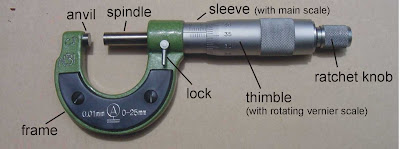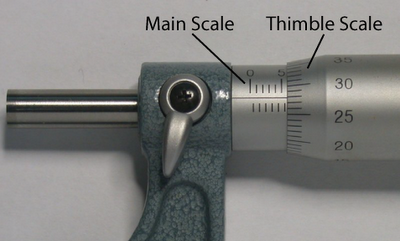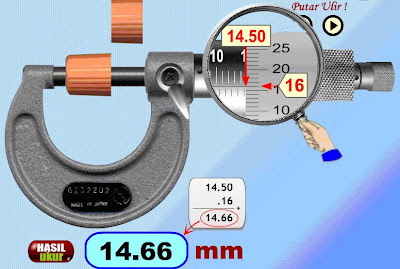## Tuesday, 27 March 2012

### Measurements in Science

Understanding Measurements

1.      Nature of Measurement: To measure a physical quantity is to make an acceptable estimate of the true and actual value of the quantity.

2.      All measurements in science are Man’s attempts to determine the true and actual value of the relevant physical quantities.

3.      Errors in Measurements
·        No measurement is without error because:
o       No measuring instruments is perfect;
o       No handling of measuring instrument (i.e. technique of measurement) is perfect; and,
o       No environment of measurement is perfectly stable or error-free.

·        An error in measurement is the difference between the actual value of the physical quantity and the value obtained in the measurement.

·        The 2 main types of errors:
Systematic Errors                                       Random Errors
-         Tend to shift all measurements             - Fluctuate from 1 measurement to the
in a particular direction - the mean         the next – the mean from measurements
from measurements is displaced            is close to the actual true value
from the actual true value
-         Are due to:                                           - Are due to:
o       Incorrect calibration of                    * Personal error e.g. parallax error
equipment – Zero errors.                * Natural errors e.g. changes in wind,
o       Improper use of equipment.               temperature, humidity, magnetic field,
o       Forgetting to account for                    gravity, etc. while experiment is on
some effects                                   * Use of wrong technique while
-         To find-out systematic errors                     taking measurements – e.g. excessive
o       Use different instrument                       pressure in turning micrometer
o       Compare data                                    screw gauge
-         To correct zero error:
o       Adjust zero adjustment screw         - To minimize random errors:
o       In the case of vernier calipers            * Take many readings & find mean
and micrometer screw gauge,            * Ensure no parallax error
Correct Reading = Reading               * Minimise natural errors
Obtained – Zero Error

(2012 P2 Q1 at pg. 346 on: Vernier calipers with zero error.)

Sensitivity, Consistency (Precision) & Accuracy

1.      Sensitivity of Measuring Instrument
·        Sensitivity of a measuring instrument is defined as the capability of that instrument 1) to respond and/or 2) to register small amounts or differences of the targeted stimuli (e.g. heat) or physical quantity (e.g. temperature) respectively.

·        Sensitivity depends on:  (2007 P1 Q3 pg. 92 - Which balance is more sensitive?)
o       The smallest division on its scale – the finer, the more sensitive (e.g. the smallest division on the thimble scale of micrometer screw gauge is 0.01mm whereas that on the vernier scale of the vernier calipers is 0.01cm);
o       The design of the instrument – e.g. the finer the capillary tube or the wall of the bulb of the thermometer, the more sensitive it is; (2011 P1 Q2 at pg. 280)
o       The choice of the responding material - e.g. alcohol is more sensitive (expands more) to heat than mercury but it is colourless.

·        Generally, measurements of large quantities do not require sensitive measuring instrument. It is in the measurements of small quantities that sensitive instruments are needed or the margins of errors can be significant.

2.      Consistency (Precision) in Measurement
·        Measurements are said to be consistent when the values of the measurements are close to each other – meaning, the deviation (i.e. the difference) of each measured value from the mean value (of all measured values) is small and the spread (i.e. the difference between the 2 outermost values) is small. (2005 P1 Q2 Pg 3) / (2008 P1 Q2 Pg. 140)

·        Therefore, high consistency means:
o       Small deviation from the mean value; and,
o       Small spread between the 2 outmost values.

·        Consistent measurements are considered precise but not necessarily accurate – they are only accurate if their mean value is close to the true and actual value.

·        Consistent measuring instrument is one with ability to register the same or nearly the same readings when a measurement is made repeatedly.

·        To improve consistency:
o       Avoid parallax errors;
o       Exercise greater care and consistency in taking readings;
o       Avoid using defective measuring instrument

3.      Accuracy in Measurement
·        Accuracy is the degree of how close a measurement is to the true and actual value of the physical quantity.

·        When repeated readings are involved, all the values of the measurement must be consistent and close to the true and actual value:
o       The mean value must be almost the same as the actual value;
o       The deviation from the mean value is small and
o       The spread is also small.

·        No measurement or measuring instrument is 100% accurate.

·        An error represents the difference between the measured value and the actual value. High accuracy means small error.

·        To improve accuracy:
o       when small values are to be measured:
·        use sensitive instrument
·        use technique which minimizes starting and ending errors – e.g. instead of measuring the time for just 1 oscillation, measure time for say 20 oscillations and then divide this time by 20;
o       take repeated readings to get average value to minimize random errors
o       avoid parallax error
o       avoid or adjust for ‘zero errors’

4.      Measurement of Length
·        There are different instruments to measure length: measuring tape, metre-rule, vernier calipers, micrometer screw gauge.

·        The choice of the instrument depends on
o       The size of the length to be measured; and,
o       The accuracy needed (or, the margin of error allowed):
Accuracy                Length to be Measured Instrument
0.01 mm                 0.1 mm ~ 2.5 cm                      Micrometer screw g.
0.01 cm (0.1mm)    1 cm ~ 12 cm diameter Vernier calipers
0.1 cm (1mm)         Several cm ~ 100 cm                Metre rule
1 cm (10mm)          Several metres                          Measuring tape

·        At Form 4 level, you should be familiar with the use of vernier calipers (2011 P1 Q1 at pg. 280) and micrometer screw gauge – verify your proficiency (2005 Paper 1 Q1 at Pg 3). You should be able to deal with zero errors in both instruments – verify by attempting questions on this.

### Micrometer Screw GaugeRatchet exerts correct amount of pressure on the object to be measured.How to take reading from micrometer screw gauge?5.      Measurement of Time Interval
·        Time interval is measured by using stopwatch.

·        There are 2 types of stopwatches depending of the accuracy needed:
Accuracy Needed              Type of Stopwatch Used
0.1 s ~ 0.2 s                       Analogue (mechanically-operated)
0.01 s                                 Digital (electronically-operated)

·        When small time interval is to be measured and the digital stopwatch is not available (say, when the period of oscillation of a pendulum is to be measured), higher accuracy or lower margin of error can be achieved by measuring the time for, say, 20 oscillations 2 or 3 times (instead of measuring the time for just 1 complete oscillation). Then take the average time for 20 oscillations and divide it by 20 to give the mean period for 1 complete oscillation.

(2010 Paper 2 Q1 at Pg. 246)

6.      Measurement of Temperature
·        There are a number of instruments to measure temperature. But the most commonly used in laboratory is the mercury thermometer.

·        There are 2 types of mercury thermometers used in laboratory:
Accuracy Needed              Temperature Range of Thermometer
1` C                                   -10` C ~ 110` C
2` C                                   0` C ~ 360` C

·        To measure body temperature, there is another mercury thermometer with the range of 35` C – 42` C. A temperature above 37` C means having fever.

·        Both mercury and alcohol expand uniformly with temperature changes. In fact, alcohol is more sensitive to heat changes than mercury. However, alcohol is colourless whereas mercury is not which is why mercury is used in thermometer.

·        Other functional features of thermometer are:
o       the thin-walled bulb which allows for quick heat transfer between heat source and the mercury for its expansion;
o       the small-diameter of the capillary tube which amplifies small expansion of mercury in the bulb into large linear expansion along the fine calibrated capillary tube allowing changes in temperature to be measured.

7.      Measurement of Electric Current & Voltage
·        Electric current (I) flowing through a point in an electric circuit is measured by the instrument ammeter or milliammeter. Both have scales calibrated in SI unit for electric current, I - ampere (A). (2005 P2 Q1 Pg. 16)

·        The choice between ammeter and milliammeter depends on the size of the current to be measured and the accuracy needed:
Current Size                       Accuracy                                  Instrument
Small (less than 1 A)           0.1 mA ~ 0.2 mA                     Milliammeter
1 A ~ a few A                    0.1 A ~ 0.2 A                          Ammeter

·        Ammeter or milliammeter is connected in series at a point in the circuit – draw diagram.

--------------------------------
·        Voltage, V, (or potential difference) between any two points in an electric circuit is measured by a voltmeter which is connected in parallel across the two points in the circuit.

·        Voltmeter has accuracy of 0.1 V ~ 0.2 V.

---------------------------------
Both the ammeter and voltmeter may have anti-parallax mirror to minimise parallax error.

---------------------------------
·        Resistance (R) of a resistor in an electric circuit is found by using Ohm’s law where R = V/I. Hence, to find the resistance of a resistor in an electric circuit, we measure both the current flowing through the resistor and the potential difference or voltage across the resistor; we then use Ohm’s Law (R = V/I) to calculate the size of the resistance in ohms.

----------------------------------------------------------------------

Segment Review Questions

1.         All measurements in science are Man’s attempts to make an acceptable estimate of the true and actual value of a physical quantity – True or False?

2.        The difference between the true and actual value of a physical quantity and the value obtained in a measurement is known as ________________.

3.        There are two main types of errors. State them, describe their differences and how each type of error may be minimised or avoided – give examples where appropriate.

4.        Attempts the following past year SPM questions:

a.         2007 P1 Q3 pg. 92 - Which balance is more sensitive?
b.         2011 P1 Q2 at pg. 280 on sensitivity in measurement
c.         2005 P1 Q2 Pg 3 on consistency and precision in measurement
d.         2008 P1 Q2 Pg. 140 – consistency and precision
e.         2011 P1 Q1 at pg. 280 – on use of vernier calipers
f.           2005 Paper 1 Q1 at Pg 3 – on use of micrometer srew gauge
g.         2010 Paper 2 Q1 at Pg. 246 – on use of stopwatch to measure 20 oscillations of pendulum
h.         2005 P2 Q1 Pg. 16 – on use of ammeter, anti-parallax mirror, etc.

-----------------------------------------------------------------
Updated on 24/01/2013 by tutortan1@gmail.com